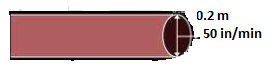# A farmer connects a pipe of internal diameter 20 cm form a canal into a cylindrical tank in her field, which is 10 m in diameter and 2 m deep. If water flows through the pipe at the rate of 3 kilometers per hour, in how much time will the tank be filled?Given

Radius of Cylindrical tank (r) = 5 m

Depth of Cylindrical tank (h) = 2 m

Speed of water flows in pipe = 3 km/hr = (3,000 m/hr)

Find out

We have to find the time taken to fill the tank

Solution

volume of water flows in 1 hr = Π × 10 × 10 × 300000 (cm3)

volume of cylindrical tank =Π × 500 × 500 × 200

time taken to be fill the tank = volume of cylindrical tank÷volume of water flow in 1 hr

t × (30π) = πr2h

t × (30π) = π × 5× 2

t = 5x5x2/30

t = 5/3 hours

t = 5/3× 60 mins

t= 100 minutes

The time required to fill the tank =100 minutes